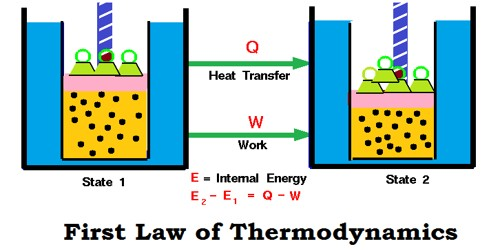# Explain Limitation of first Law of Thermodynamics

The first law of thermodynamics tells us that energy can be changed from one form to another but can be neither created nor destroyed in any process. This law states that the total energy of an isolated system is constant. Energy can be transformed from one form to another, but can neither be created nor destroyed. However, the first law fails to give the feasibility of the process or change of state that the system undergoes. For instance, the first law fails to explain why heat flows from hot end to cold end when a metallic rod is heated at one end and not on other and vice-versa.

The mathematical expression which is used to represent the first law of thermodynamics is ΔE = q-w, where ΔE refers to the internal energy change of the system when ‘w’ amount of work is done by the system when it absorbs ‘q’ amount of heat and carries out ‘w’ amount of work.

However, this law possesses many limitations such as given below.

1. The first law of thermodynamics merely indicates that in any process there is a transformation between the various forms of energy involved in the process but provides no information regarding the feasibility of such transformation.
2. The first law does not provide any information regarding the direction a process will take whether it is a spontaneous or a non-spontaneous process.
3. The reverse procedure is not possible. In real practice, the heat doesn’t convert totally into work. If it would have been possible to convert the whole heat into work, then we could drive ships across the ocean by extracting heat from the water of the ocean.
4. It does not explain anything about the direction of the flow of heat. The first law does not indicate whether heat can flow from a cold end to a hot end or not. For example – we cannot extract heat from the ice by cooling it to a low temperature. Practically it is not possible to convert the heat energy into an equivalent amount of work.
5. The first law does not denote that procedure is feasible or not for example: when a rod is heated at one end then equilibrium has to be obtained which is possible only by some expenditure of energy.